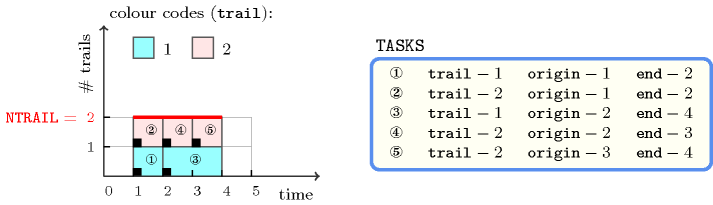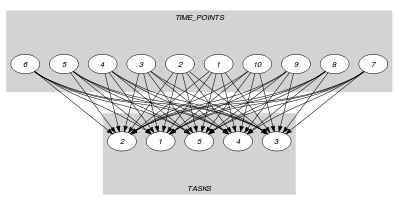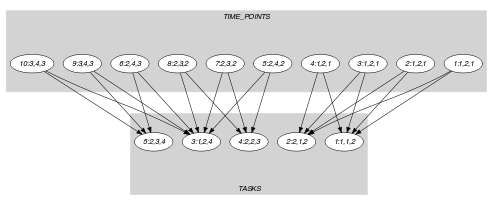## 5.402. track

Origin
Constraint

$\mathrm{𝚝𝚛𝚊𝚌𝚔}\left(\mathrm{𝙽𝚃𝚁𝙰𝙸𝙻},\mathrm{𝚃𝙰𝚂𝙺𝚂}\right)$

Arguments
 $\mathrm{𝙽𝚃𝚁𝙰𝙸𝙻}$ $\mathrm{𝚒𝚗𝚝}$ $\mathrm{𝚃𝙰𝚂𝙺𝚂}$ $\mathrm{𝚌𝚘𝚕𝚕𝚎𝚌𝚝𝚒𝚘𝚗}\left(\mathrm{𝚝𝚛𝚊𝚒𝚕}-\mathrm{𝚒𝚗𝚝},\mathrm{𝚘𝚛𝚒𝚐𝚒𝚗}-\mathrm{𝚍𝚟𝚊𝚛},\mathrm{𝚎𝚗𝚍}-\mathrm{𝚍𝚟𝚊𝚛}\right)$
Restrictions
 $\mathrm{𝙽𝚃𝚁𝙰𝙸𝙻}>0$ $\mathrm{𝙽𝚃𝚁𝙰𝙸𝙻}\le |\mathrm{𝚃𝙰𝚂𝙺𝚂}|$ $|\mathrm{𝚃𝙰𝚂𝙺𝚂}|>0$ $\mathrm{𝚛𝚎𝚚𝚞𝚒𝚛𝚎𝚍}$$\left(\mathrm{𝚃𝙰𝚂𝙺𝚂},\left[\mathrm{𝚝𝚛𝚊𝚒𝚕},\mathrm{𝚘𝚛𝚒𝚐𝚒𝚗},\mathrm{𝚎𝚗𝚍}\right]\right)$ $\mathrm{𝚃𝙰𝚂𝙺𝚂}.\mathrm{𝚘𝚛𝚒𝚐𝚒𝚗}\le \mathrm{𝚃𝙰𝚂𝙺𝚂}.\mathrm{𝚎𝚗𝚍}$
Purpose

The $\mathrm{𝚝𝚛𝚊𝚌𝚔}$ constraint forces that, at each point in time overlapped by at least one task, the number of distinct values of the $\mathrm{𝚝𝚛𝚊𝚒𝚕}$ attribute of the set of tasks that overlap that point, is equal to $\mathrm{𝙽𝚃𝚁𝙰𝙸𝙻}$.

Example
$\left(\begin{array}{c}2,〈\begin{array}{ccc}\mathrm{𝚝𝚛𝚊𝚒𝚕}-1\hfill & \mathrm{𝚘𝚛𝚒𝚐𝚒𝚗}-1\hfill & \mathrm{𝚎𝚗𝚍}-2,\hfill \\ \mathrm{𝚝𝚛𝚊𝚒𝚕}-2\hfill & \mathrm{𝚘𝚛𝚒𝚐𝚒𝚗}-1\hfill & \mathrm{𝚎𝚗𝚍}-2,\hfill \\ \mathrm{𝚝𝚛𝚊𝚒𝚕}-1\hfill & \mathrm{𝚘𝚛𝚒𝚐𝚒𝚗}-2\hfill & \mathrm{𝚎𝚗𝚍}-4,\hfill \\ \mathrm{𝚝𝚛𝚊𝚒𝚕}-2\hfill & \mathrm{𝚘𝚛𝚒𝚐𝚒𝚗}-2\hfill & \mathrm{𝚎𝚗𝚍}-3,\hfill \\ \mathrm{𝚝𝚛𝚊𝚒𝚕}-2\hfill & \mathrm{𝚘𝚛𝚒𝚐𝚒𝚗}-3\hfill & \mathrm{𝚎𝚗𝚍}-4\hfill \end{array}〉\hfill \end{array}\right)$

Figure 5.402.1 represents the tasks of the example: to the ${i}^{th}$ task of the $\mathrm{𝚃𝙰𝚂𝙺𝚂}$ collection corresponds a rectangle labelled by $i$. The $\mathrm{𝚝𝚛𝚊𝚌𝚔}$ constraint holds since:

• The first and second tasks both overlap instant 1 and have a respective trail of 1 and 2. This makes two distinct values for the trail attribute at instant 1.

• The third and fourth tasks both overlap instant 2 and have a respective trail of 1 and 2. This makes two distinct values for the trail attribute at instant 2.

• The third and fifth tasks both overlap instant 3 and have a respective trail of 1 and 2. This makes two distinct values for the trail attribute at instant 3.

##### Figure 5.402.1. The tasks associated with the example of the Example slot, at each instant we have two distinct values for the $\mathrm{𝚝𝚛𝚊𝚒𝚕}$ attribute ($\mathrm{𝙽𝚃𝚁𝙰𝙸𝙻}=2$)Typical
 $\mathrm{𝙽𝚃𝚁𝙰𝙸𝙻}<|\mathrm{𝚃𝙰𝚂𝙺𝚂}|$ $|\mathrm{𝚃𝙰𝚂𝙺𝚂}|>1$ $\mathrm{𝚛𝚊𝚗𝚐𝚎}$$\left(\mathrm{𝚃𝙰𝚂𝙺𝚂}.\mathrm{𝚝𝚛𝚊𝚒𝚕}\right)>1$ $\mathrm{𝚃𝙰𝚂𝙺𝚂}.\mathrm{𝚘𝚛𝚒𝚐𝚒𝚗}<\mathrm{𝚃𝙰𝚂𝙺𝚂}.\mathrm{𝚎𝚗𝚍}$
Symmetries
• Items of $\mathrm{𝚃𝙰𝚂𝙺𝚂}$ are permutable.

• All occurrences of two distinct values of $\mathrm{𝚃𝙰𝚂𝙺𝚂}.\mathrm{𝚝𝚛𝚊𝚒𝚕}$ can be swapped; all occurrences of a value of $\mathrm{𝚃𝙰𝚂𝙺𝚂}.\mathrm{𝚝𝚛𝚊𝚒𝚕}$ can be renamed to any unused value.

• One and the same constant can be added to the $\mathrm{𝚘𝚛𝚒𝚐𝚒𝚗}$ and $\mathrm{𝚎𝚗𝚍}$ attributes of all items of $\mathrm{𝚃𝙰𝚂𝙺𝚂}$.

Reformulation

The $\mathrm{𝚝𝚛𝚊𝚌𝚔}$ constraint can be expressed in term of a set of reified constraints and of $2·|\mathrm{𝚃𝙰𝚂𝙺𝚂}|$ $\mathrm{𝚗𝚟𝚊𝚕𝚞𝚎}$ constraints:

1. For each pair of tasks $\mathrm{𝚃𝙰𝚂𝙺𝚂}\left[i\right],\mathrm{𝚃𝙰𝚂𝙺𝚂}\left[j\right]$ $\left(i,j\in \left[1,|\mathrm{𝚃𝙰𝚂𝙺𝚂}|\right]\right)$ of the $\mathrm{𝚃𝙰𝚂𝙺𝚂}$ collection we create a variable ${T}_{ij}^{\mathrm{𝚘𝚛𝚒𝚐𝚒𝚗}}$ which is set to the $\mathrm{𝚝𝚛𝚊𝚒𝚕}$ attribute of task $\mathrm{𝚃𝙰𝚂𝙺𝚂}\left[j\right]$ if task $\mathrm{𝚃𝙰𝚂𝙺𝚂}\left[j\right]$ overlaps the origin attribute of task $\mathrm{𝚃𝙰𝚂𝙺𝚂}\left[i\right]$, and to the $\mathrm{𝚝𝚛𝚊𝚒𝚕}$ attribute of task $\mathrm{𝚃𝙰𝚂𝙺𝚂}\left[i\right]$ otherwise:

• If $i=j$:

• ${T}_{ij}^{\mathrm{𝚘𝚛𝚒𝚐𝚒𝚗}}=\mathrm{𝚃𝙰𝚂𝙺𝚂}\left[i\right].\mathrm{𝚝𝚛𝚊𝚒𝚕}$.

• If $i\ne j$:

• ${T}_{ij}^{\mathrm{𝚘𝚛𝚒𝚐𝚒𝚗}}=\mathrm{𝚃𝙰𝚂𝙺𝚂}\left[i\right].\mathrm{𝚝𝚛𝚊𝚒𝚕}\vee {T}_{ij}^{\mathrm{𝚘𝚛𝚒𝚐𝚒𝚗}}=\mathrm{𝚃𝙰𝚂𝙺𝚂}\left[j\right].\mathrm{𝚝𝚛𝚊𝚒𝚕}$.

• $\left(\left(\mathrm{𝚃𝙰𝚂𝙺𝚂}\left[j\right].\mathrm{𝚘𝚛𝚒𝚐𝚒𝚗}\le \mathrm{𝚃𝙰𝚂𝙺𝚂}\left[i\right].\mathrm{𝚘𝚛𝚒𝚐𝚒𝚗}\wedge$

$\mathrm{𝚃𝙰𝚂𝙺𝚂}\left[j\right].\mathrm{𝚎𝚗𝚍}>\mathrm{𝚃𝙰𝚂𝙺𝚂}\left[i\right].\mathrm{𝚘𝚛𝚒𝚐𝚒𝚗}\right)\wedge \left({T}_{ij}^{\mathrm{𝚘𝚛𝚒𝚐𝚒𝚗}}=\mathrm{𝚃𝙰𝚂𝙺𝚂}\left[j\right].\mathrm{𝚝𝚛𝚊𝚒𝚕}\right)\right)\vee$

$\left(\left(\mathrm{𝚃𝙰𝚂𝙺𝚂}\left[j\right].\mathrm{𝚘𝚛𝚒𝚐𝚒𝚗}>\mathrm{𝚃𝙰𝚂𝙺𝚂}\left[i\right].\mathrm{𝚘𝚛𝚒𝚐𝚒𝚗}\vee$

$\mathrm{𝚃𝙰𝚂𝙺𝚂}\left[j\right].\mathrm{𝚎𝚗𝚍}\le \mathrm{𝚃𝙰𝚂𝙺𝚂}\left[i\right].\mathrm{𝚘𝚛𝚒𝚐𝚒𝚗}\right)\wedge \left({T}_{ij}^{\mathrm{𝚘𝚛𝚒𝚐𝚒𝚗}}=\mathrm{𝚃𝙰𝚂𝙺𝚂}\left[i\right].\mathrm{𝚝𝚛𝚊𝚒𝚕}\right)\right)$

2. For each task $\mathrm{𝚃𝙰𝚂𝙺𝚂}\left[i\right]$ $\left(i\in \left[1,|\mathrm{𝚃𝙰𝚂𝙺𝚂}|\right]\right)$ we impose the number of distinct trails associated with the tasks that overlap the origin of task $\mathrm{𝚃𝙰𝚂𝙺𝚂}\left[i\right]$ ($\mathrm{𝚃𝙰𝚂𝙺𝚂}\left[i\right]$ overlaps its own origin) to be equal to $\mathrm{𝙽𝚃𝚁𝙰𝙸𝙻}$:

$\mathrm{𝚗𝚟𝚊𝚕𝚞𝚎}$$\left(\mathrm{𝙽𝚃𝚁𝙰𝙸𝙻},〈{T}_{i1}^{\mathrm{𝚘𝚛𝚒𝚐𝚒𝚗}},{T}_{i2}^{\mathrm{𝚘𝚛𝚒𝚐𝚒𝚗}},\cdots ,{T}_{i|\mathrm{𝚃𝙰𝚂𝙺𝚂}|}^{\mathrm{𝚘𝚛𝚒𝚐𝚒𝚗}}〉\right)$.

3. For each pair of tasks $\mathrm{𝚃𝙰𝚂𝙺𝚂}\left[i\right],\mathrm{𝚃𝙰𝚂𝙺𝚂}\left[j\right]$ $\left(i,j\in \left[1,|\mathrm{𝚃𝙰𝚂𝙺𝚂}|\right]\right)$ of the $\mathrm{𝚃𝙰𝚂𝙺𝚂}$ collection we create a variable ${T}_{ij}^{\mathrm{𝚎𝚗𝚍}}$ which is set to the $\mathrm{𝚝𝚛𝚊𝚒𝚕}$ attribute of task $\mathrm{𝚃𝙰𝚂𝙺𝚂}\left[j\right]$ if task $\mathrm{𝚃𝙰𝚂𝙺𝚂}\left[j\right]$ overlaps the end attribute of task $\mathrm{𝚃𝙰𝚂𝙺𝚂}\left[i\right]$, and to the $\mathrm{𝚝𝚛𝚊𝚒𝚕}$ attribute of task $\mathrm{𝚃𝙰𝚂𝙺𝚂}\left[i\right]$ otherwise:

• If $i=j$:

• ${T}_{ij}^{\mathrm{𝚎𝚗𝚍}}=\mathrm{𝚃𝙰𝚂𝙺𝚂}\left[i\right].\mathrm{𝚝𝚛𝚊𝚒𝚕}$.

• If $i\ne j$:

• ${T}_{ij}^{\mathrm{𝚎𝚗𝚍}}=\mathrm{𝚃𝙰𝚂𝙺𝚂}\left[i\right].\mathrm{𝚝𝚛𝚊𝚒𝚕}\vee {T}_{ij}^{\mathrm{𝚎𝚗𝚍}}=\mathrm{𝚃𝙰𝚂𝙺𝚂}\left[j\right].\mathrm{𝚝𝚛𝚊𝚒𝚕}$.

• $\left(\left(\mathrm{𝚃𝙰𝚂𝙺𝚂}\left[j\right].\mathrm{𝚘𝚛𝚒𝚐𝚒𝚗}\le \mathrm{𝚃𝙰𝚂𝙺𝚂}\left[i\right].\mathrm{𝚎𝚗𝚍}-1\wedge$

$\mathrm{𝚃𝙰𝚂𝙺𝚂}\left[j\right].\mathrm{𝚎𝚗𝚍}>\mathrm{𝚃𝙰𝚂𝙺𝚂}\left[i\right].\mathrm{𝚎𝚗𝚍}-1\right)\wedge \left({T}_{ij}^{\mathrm{𝚎𝚗𝚍}}=\mathrm{𝚃𝙰𝚂𝙺𝚂}\left[j\right].\mathrm{𝚝𝚛𝚊𝚒𝚕}\right)\right)\vee$

$\left(\left(\mathrm{𝚃𝙰𝚂𝙺𝚂}\left[j\right].\mathrm{𝚘𝚛𝚒𝚐𝚒𝚗}>\mathrm{𝚃𝙰𝚂𝙺𝚂}\left[i\right].\mathrm{𝚎𝚗𝚍}-1\vee$

$\mathrm{𝚃𝙰𝚂𝙺𝚂}\left[j\right].\mathrm{𝚎𝚗𝚍}\le \mathrm{𝚃𝙰𝚂𝙺𝚂}\left[i\right].\mathrm{𝚎𝚗𝚍}-1\right)\wedge \left({T}_{ij}^{\mathrm{𝚎𝚗𝚍}}=\mathrm{𝚃𝙰𝚂𝙺𝚂}\left[i\right].\mathrm{𝚝𝚛𝚊𝚒𝚕}\right)\right)$

4. For each task $\mathrm{𝚃𝙰𝚂𝙺𝚂}\left[i\right]$ $\left(i\in \left[1,|\mathrm{𝚃𝙰𝚂𝙺𝚂}|\right]\right)$ we impose the number of distinct trails associated with the tasks that overlap the end of task $\mathrm{𝚃𝙰𝚂𝙺𝚂}\left[i\right]$ ($\mathrm{𝚃𝙰𝚂𝙺𝚂}\left[i\right]$ overlaps its own end) to be equal to $\mathrm{𝙽𝚃𝚁𝙰𝙸𝙻}$:

$\mathrm{𝚗𝚟𝚊𝚕𝚞𝚎}$$\left(\mathrm{𝙽𝚃𝚁𝙰𝙸𝙻},〈{T}_{i1}^{\mathrm{𝚎𝚗𝚍}},{T}_{i2}^{\mathrm{𝚎𝚗𝚍}},\cdots ,{T}_{i|\mathrm{𝚃𝙰𝚂𝙺𝚂}|}^{\mathrm{𝚎𝚗𝚍}}〉\right)$.

With respect to the Example slot we get the following conjunction of $\mathrm{𝚗𝚟𝚊𝚕𝚞𝚎}$ constraints:

Keywords
Derived Collection
$\mathrm{𝚌𝚘𝚕}\left(\begin{array}{c}\mathrm{𝚃𝙸𝙼𝙴}_\mathrm{𝙿𝙾𝙸𝙽𝚃𝚂}-\mathrm{𝚌𝚘𝚕𝚕𝚎𝚌𝚝𝚒𝚘𝚗}\left(\begin{array}{c}\mathrm{𝚘𝚛𝚒𝚐𝚒𝚗}-\mathrm{𝚍𝚟𝚊𝚛},\hfill \\ \mathrm{𝚎𝚗𝚍}-\mathrm{𝚍𝚟𝚊𝚛},\hfill \\ \mathrm{𝚙𝚘𝚒𝚗𝚝}-\mathrm{𝚍𝚟𝚊𝚛}\hfill \end{array}\right),\hfill \\ \left[\begin{array}{c}\mathrm{𝚒𝚝𝚎𝚖}\left(\begin{array}{c}\mathrm{𝚘𝚛𝚒𝚐𝚒𝚗}-\mathrm{𝚃𝙰𝚂𝙺𝚂}.\mathrm{𝚘𝚛𝚒𝚐𝚒𝚗},\hfill \\ \mathrm{𝚎𝚗𝚍}-\mathrm{𝚃𝙰𝚂𝙺𝚂}.\mathrm{𝚎𝚗𝚍},\hfill \\ \mathrm{𝚙𝚘𝚒𝚗𝚝}-\mathrm{𝚃𝙰𝚂𝙺𝚂}.\mathrm{𝚘𝚛𝚒𝚐𝚒𝚗}\hfill \end{array}\right),\hfill \\ \mathrm{𝚒𝚝𝚎𝚖}\left(\begin{array}{c}\mathrm{𝚘𝚛𝚒𝚐𝚒𝚗}-\mathrm{𝚃𝙰𝚂𝙺𝚂}.\mathrm{𝚘𝚛𝚒𝚐𝚒𝚗},\hfill \\ \mathrm{𝚎𝚗𝚍}-\mathrm{𝚃𝙰𝚂𝙺𝚂}.\mathrm{𝚎𝚗𝚍},\hfill \\ \mathrm{𝚙𝚘𝚒𝚗𝚝}-\mathrm{𝚃𝙰𝚂𝙺𝚂}.\mathrm{𝚎𝚗𝚍}-1\hfill \end{array}\right)\hfill \end{array}\right]\hfill \end{array}\right)$
Arc input(s)

$\mathrm{𝚃𝙰𝚂𝙺𝚂}$

Arc generator
$\mathrm{𝑆𝐸𝐿𝐹}$$↦\mathrm{𝚌𝚘𝚕𝚕𝚎𝚌𝚝𝚒𝚘𝚗}\left(\mathrm{𝚝𝚊𝚜𝚔𝚜}\right)$

Arc arity
Arc constraint(s)
$\mathrm{𝚝𝚊𝚜𝚔𝚜}.\mathrm{𝚘𝚛𝚒𝚐𝚒𝚗}\le \mathrm{𝚝𝚊𝚜𝚔𝚜}.\mathrm{𝚎𝚗𝚍}$
Graph property(ies)
$\mathrm{𝐍𝐀𝐑𝐂}$$=|\mathrm{𝚃𝙰𝚂𝙺𝚂}|$

Arc input(s)

$\mathrm{𝚃𝙸𝙼𝙴}_\mathrm{𝙿𝙾𝙸𝙽𝚃𝚂}$ $\mathrm{𝚃𝙰𝚂𝙺𝚂}$

Arc generator
$\mathrm{𝑃𝑅𝑂𝐷𝑈𝐶𝑇}$$↦\mathrm{𝚌𝚘𝚕𝚕𝚎𝚌𝚝𝚒𝚘𝚗}\left(\mathrm{𝚝𝚒𝚖𝚎}_\mathrm{𝚙𝚘𝚒𝚗𝚝𝚜},\mathrm{𝚝𝚊𝚜𝚔𝚜}\right)$

Arc arity
Arc constraint(s)
 $•\mathrm{𝚝𝚒𝚖𝚎}_\mathrm{𝚙𝚘𝚒𝚗𝚝𝚜}.\mathrm{𝚎𝚗𝚍}>\mathrm{𝚝𝚒𝚖𝚎}_\mathrm{𝚙𝚘𝚒𝚗𝚝𝚜}.\mathrm{𝚘𝚛𝚒𝚐𝚒𝚗}$ $•\mathrm{𝚝𝚊𝚜𝚔𝚜}.\mathrm{𝚘𝚛𝚒𝚐𝚒𝚗}\le \mathrm{𝚝𝚒𝚖𝚎}_\mathrm{𝚙𝚘𝚒𝚗𝚝𝚜}.\mathrm{𝚙𝚘𝚒𝚗𝚝}$ $•\mathrm{𝚝𝚒𝚖𝚎}_\mathrm{𝚙𝚘𝚒𝚗𝚝𝚜}.\mathrm{𝚙𝚘𝚒𝚗𝚝}<\mathrm{𝚝𝚊𝚜𝚔𝚜}.\mathrm{𝚎𝚗𝚍}$
Sets
$\begin{array}{c}\mathrm{𝖲𝖴𝖢𝖢}↦\hfill \\ \left[\begin{array}{c}\mathrm{𝚜𝚘𝚞𝚛𝚌𝚎},\hfill \\ \mathrm{𝚟𝚊𝚛𝚒𝚊𝚋𝚕𝚎𝚜}-\mathrm{𝚌𝚘𝚕}\left(\begin{array}{c}\mathrm{𝚅𝙰𝚁𝙸𝙰𝙱𝙻𝙴𝚂}-\mathrm{𝚌𝚘𝚕𝚕𝚎𝚌𝚝𝚒𝚘𝚗}\left(\mathrm{𝚟𝚊𝚛}-\mathrm{𝚍𝚟𝚊𝚛}\right),\hfill \\ \mathrm{𝚒𝚝𝚎𝚖}\left(\mathrm{𝚟𝚊𝚛}-\mathrm{𝚃𝙰𝚂𝙺𝚂}.\mathrm{𝚝𝚛𝚊𝚒𝚕}\right)\right]\hfill \end{array}\right)\hfill \end{array}\right]\hfill \end{array}$

Constraint(s) on sets
$\mathrm{𝚗𝚟𝚊𝚕𝚞𝚎}$$\left(\mathrm{𝙽𝚃𝚁𝙰𝙸𝙻},\mathrm{𝚟𝚊𝚛𝚒𝚊𝚋𝚕𝚎𝚜}\right)$
Graph model

Parts (A) and (B) of Figure 5.402.2 respectively show the initial and final graph of the second graph constraint of the Example slot.

##### Figure 5.402.2. Initial and final graph of the $\mathrm{𝚝𝚛𝚊𝚌𝚔}$ constraint(a)(b)
Signature

Consider the first graph constraint. Since we use the $\mathrm{𝑆𝐸𝐿𝐹}$ arc generator on the $\mathrm{𝚃𝙰𝚂𝙺𝚂}$ collection, the maximum number of arcs of the final graph is equal to $|\mathrm{𝚃𝙰𝚂𝙺𝚂}|$. Therefore we can rewrite $\mathrm{𝐍𝐀𝐑𝐂}=|\mathrm{𝚃𝙰𝚂𝙺𝚂}|$ to $\mathrm{𝐍𝐀𝐑𝐂}\ge |\mathrm{𝚃𝙰𝚂𝙺𝚂}|$ and simplify $\underline{\overline{\mathrm{𝐍𝐀𝐑𝐂}}}$ to $\overline{\mathrm{𝐍𝐀𝐑𝐂}}$.